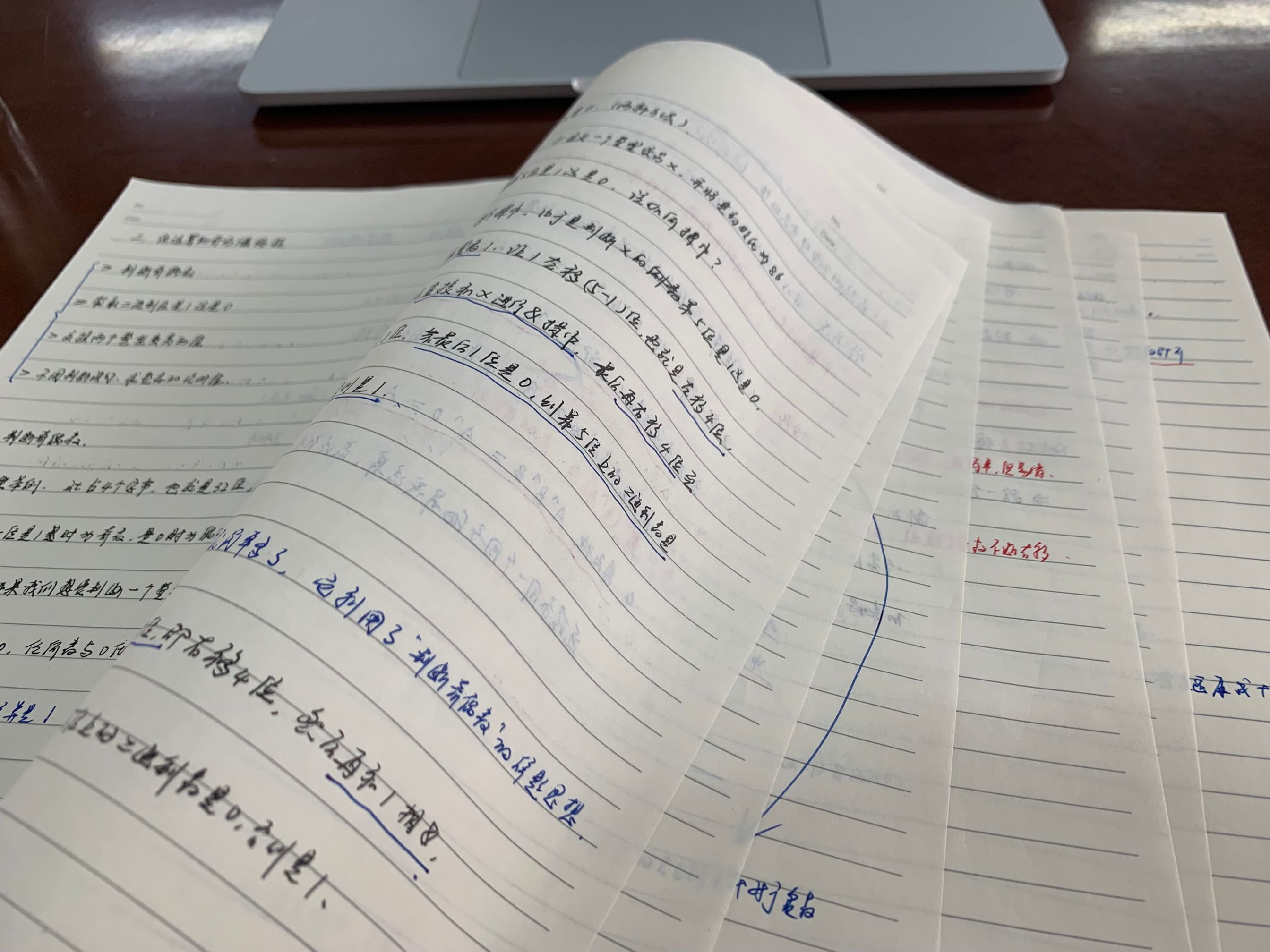WHCSRL 技术网

# 蓝桥杯算法竞赛系列第一章——位运算的奇巧淫技及其实战

1、按位与 &

2、按位或 |

3、按位取反 ~

4、按位异或 ^

5、补充了解移位运算

1.左移<<

2.右移>>

3.无符号右移>>>

6、补充了解原码反码补码

1.原码

2.反码

3.补码

1、判断奇偶数

2、判断二进制位是1还是0（两种方法）

代码执行

3、交换两个整型变量的值（异或法）

代码执行

4、不用判断语句，求整数的绝对值

【前言】：笔者是根据蓝桥杯官方发布的视频整理出的笔记，博文是由笔记二次整理而来，具有较强的针对性，打算参赛的小伙伴跟着笔者一起行动起来吧！为了方便更多的铁汁们，代码示例中笔者采用的是C语言编写。

【友情提示】：在接下来的两个半月中，博主将会持续推出两个系列的博文，一是零基础搞定C语言，二是蓝桥杯算法竞赛，包括一些刷题感悟与总结哦，当然后面还会有往年的蓝桥杯真题总结，喜欢的铁汁们可以关注笔者哦。

# 一、位运算符总结概述

## 5、补充了解移位运算

### 2.右移>>

那么对于long类型来说，超出时需要模上64（模-->求余）

## 6、补充了解原码反码补码

### 3.补码

``    int a = 20;  //整型a是4个字节，32位 	// 00000000 00000000 00000000 00010100 - 原码	// 00000000 00000000 00000000 00010100 - 反码	// 00000000 00000000 00000000 00010100 - 补码 	int b = -10; 	// 10000000 00000000 00000000 00001010 - 原码	// 11111111 11111111 11111111 11110101 - 反码	// 11111111 11111111 11111111 11110110 - 补码``

# 二、蓝桥云课：位运算的奇巧淫技

##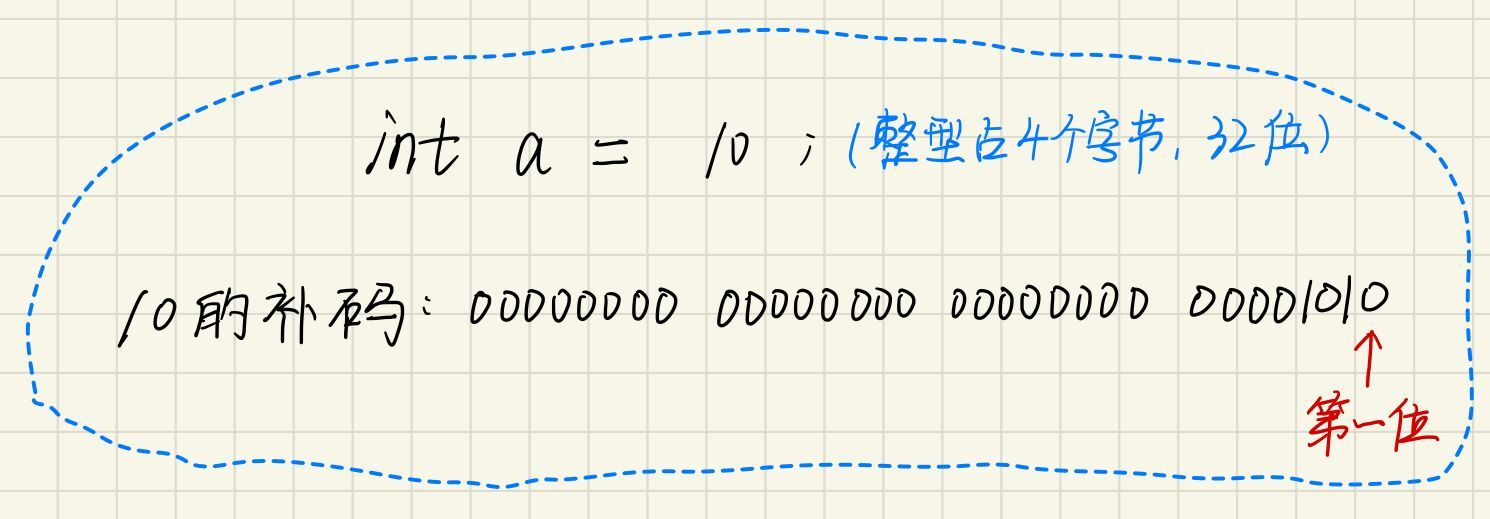### 代码执行

``````#include<stdio.h>int main(){	printf("判断奇偶数：
");	int num = 12;	if ((num & 1) == 0)	{		printf("%%%%d是偶数
",num);	}	else	{		printf("%%%%d是奇数
", num);	}	return 0;}``````

## 2、判断二进制位是1还是0（两种方法）

现需要判断第5位是1还是0， 该如何操作？

### 代码执行

``````#include<stdio.h>int main(){	int x = 86;	printf("判断整数86的二进制第5位是1还是0:
");	//运算符的使用有优先级，我们不必特意去记这个，可能存在歧义的时候，就加上括号，这样既保险又省心	if ((((1 << 4) & x) >> 4) == 0)	{		putchar('0');	}	else	{		putchar('1');	}	return 0;}``````

### 代码执行

``````#include<stdio.h>int main(){	int x = 86;	printf("判断整数86的二进制第5位是1还是0:
");	if (((x >> 4) & 1) == 0)	{		putchar('0');	}	else	{		putchar('1');	}	return 0;}``````

## 3、交换两个整型变量的值（异或法）

### 解题思路

1.交换律：可任意交换运算因子的位置，结果不变；

如：a ^ b ^ c = b ^ a ^ c;

2.结合律：即(a ^ b) ^ c == a ^ ( b ^ c) ;

3.对于任何数x, 都有x ^ x = 0, x ^ 0 = x,同自己求异或为0，同0求异或为自己

4.自反性：A ^ B ^ B = A ^ 0 = A, 连续和同一个因子做异或运算，最终结果为自己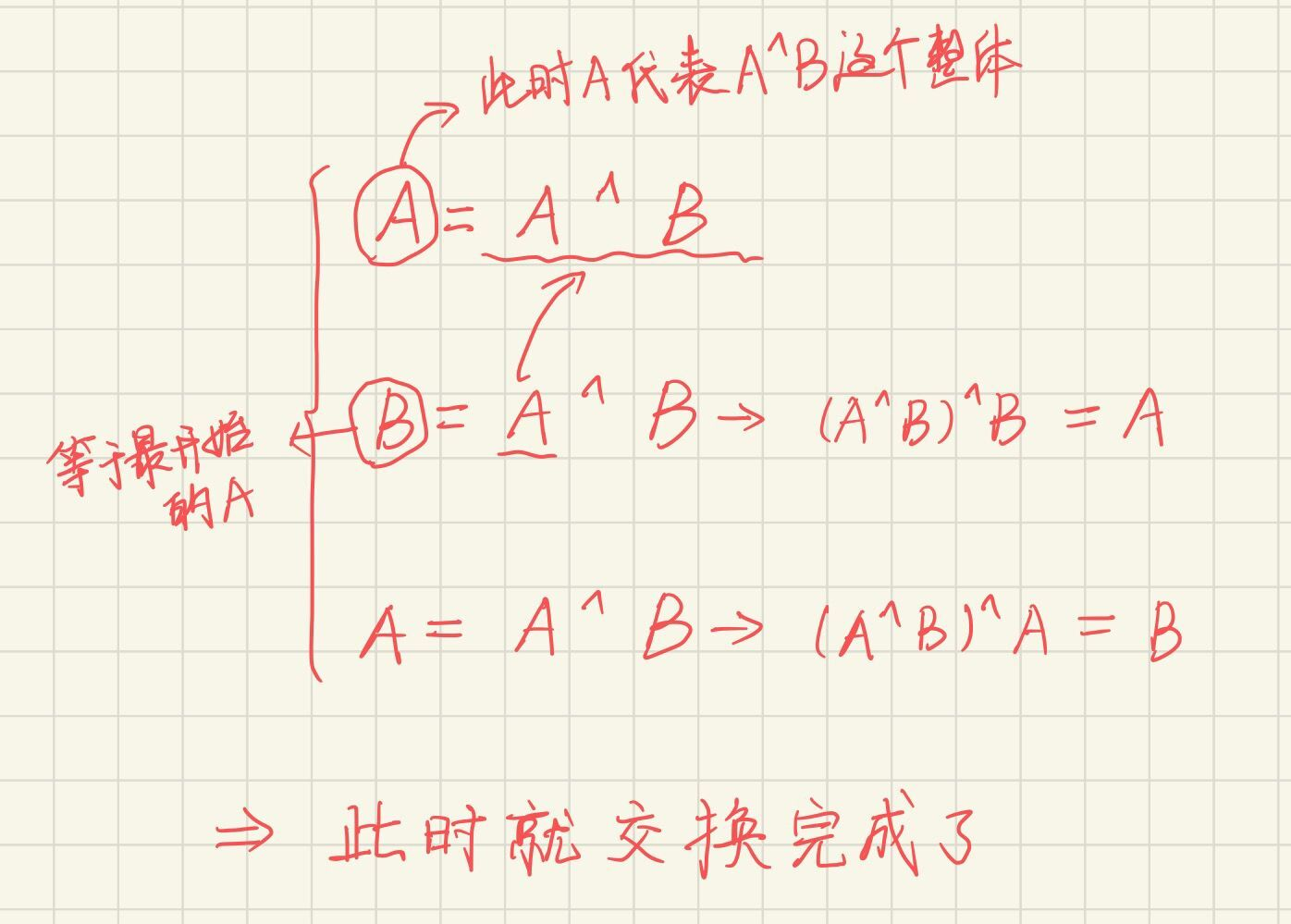### 代码执行

``````#include<stdio.h>int main(){	int A = 10;	int B = 20;	printf("交换前A = %%%%d B = %%%%d
", A, B);	A = A ^ B;	B = A ^ B;	A = A ^ B;	printf("交换后A = %%%%d B = %%%%d
", A, B);	return 0;}``````

## 4、不用判断语句，求整数的绝对值

### 解题思路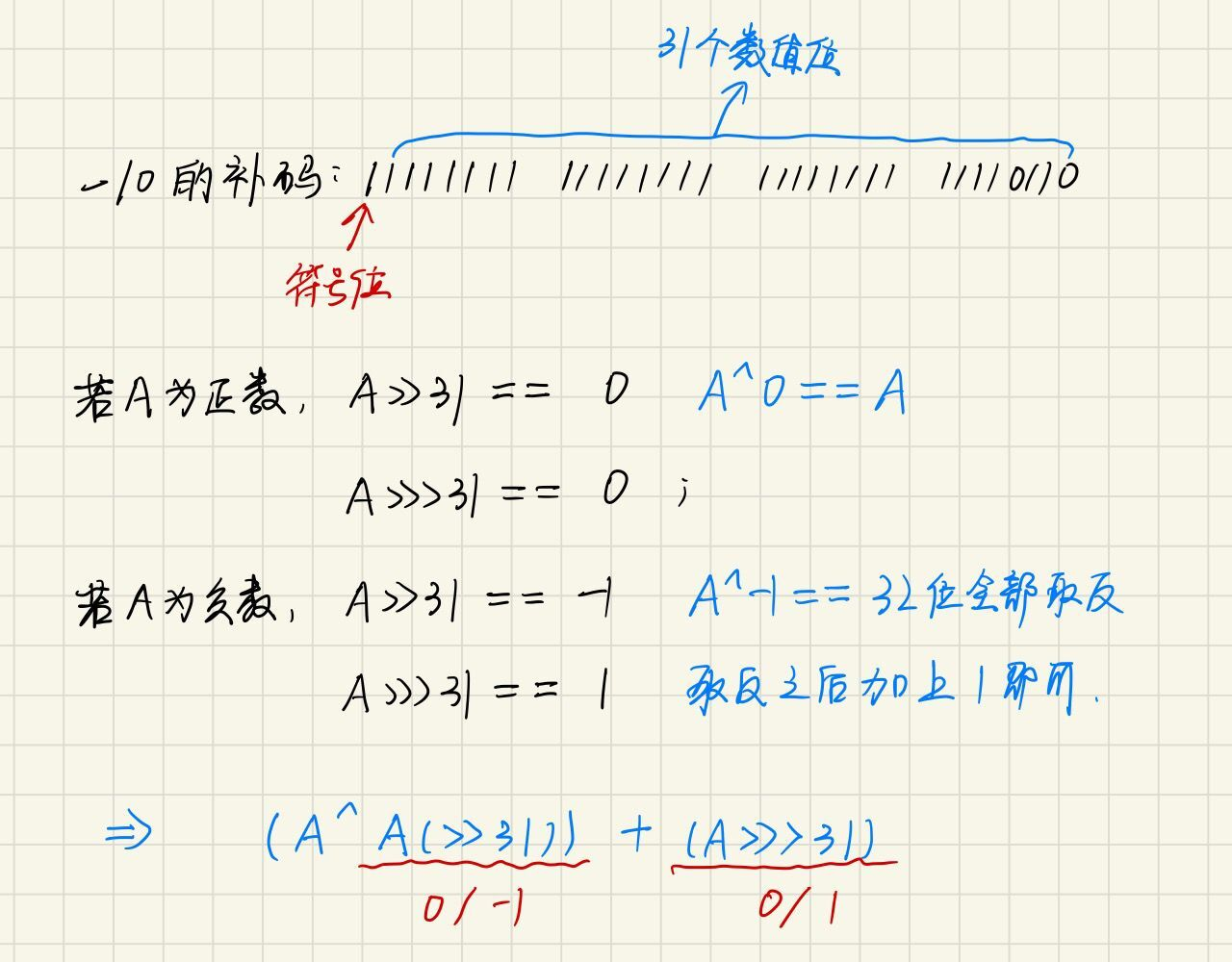### 代码执行

``public class Test10_16 {	public static void main(String[] args) { 		System.out.println("不用判断语句，求整数的绝对值");		int num5 = -100;		System.out.println("num5的绝对值是：" + ((num5 ^ (num5 >> 31)) + (num5 >>> 31)));	}}``

# 三、实战例题

## 例1、如何找数组中唯一成对的那个数

1-10这10个数放在含有11个元素的数组中，只有唯一一个元素重复，其他均只出现一次，要求每个数组元素只能够被访问一次，请设计一个算法，将它找出来，不用辅助存储空间，能否设计一个算法实现？

### 代码执行

``````#include<stdio.h>int main(){	int arr[] = { 1,2,3,2,6,4,7,5,8,9,10 };	int sz = sizeof(arr) / sizeof(arr);	int x = 0;//之所以将x初始化为0，因为0与任何数异或都是它自己	for (int i = 0; i < sz; i++)	{		x = x ^ arr[i];	}	for (int i = 1; i <= sz - 1; i++)	{		x = x ^ i;	}	printf("%%%%d
", x);		return 0;}``````

## 例2、找出落单的那个数

### 代码执行

``````#include<stdio.h>int main(){	int arr[] = { 1,1,2,3,3,4,4,5,5 };	int sz = sizeof(arr) / sizeof(arr);	int x = 0;	for (int i = 0; i < sz; i++)	{		x = x ^ arr[i];	}	printf("%%%%d
", x);	return 0;}``````

## 例3、二进制中1的个数

### 代码执行

``````#include<stdio.h>int main(){	int num = 9;	int count = 0;//用于计数	for (int i = 0; i < 32; i++)	{        //一定要注意写法，虽然简单，但是容易出错		if ((num & (1 << i)) == (1 << i))-----注意写成if((num & (1 << i) == 1)就错了		{			count++;		}	}	printf("%%%%d
", count);	return 0;}``````

### 代码执行

``````#include<stdio.h>int main(){	int num = 9;	int count = 0;	for (int i = 0; i < 32; i++)	{		if (((num >> i) & 1) == 1)		{			count++;		}	}	printf("%%%%d
", count);	return 0;}``````

### 方法3解题思路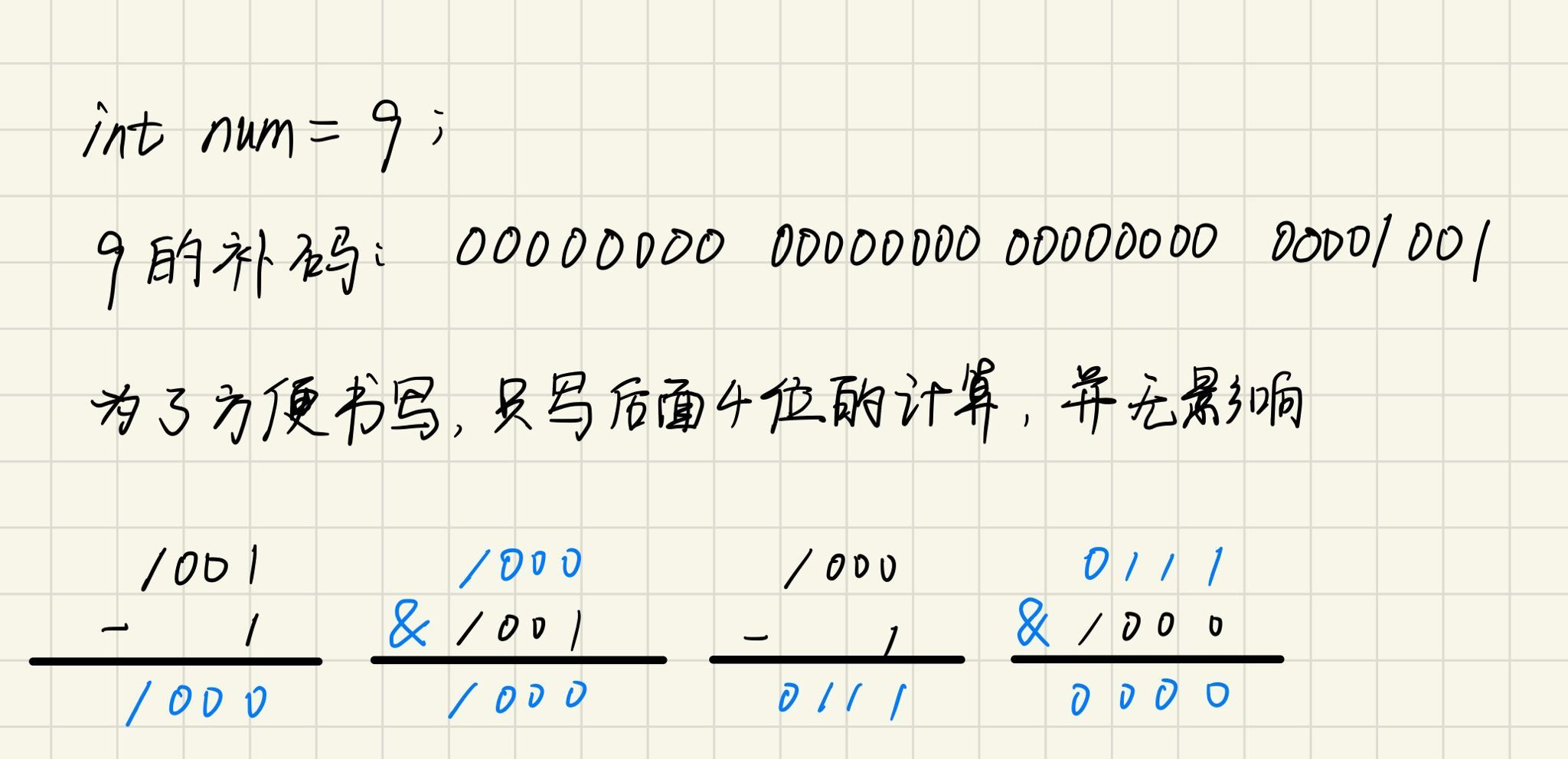可能大家一开始的时候想不到这个解法，笔者也是，我一开始看的时候也很蒙，但是当你看完了之后会有种醍醐灌顶的感觉，说明你理解了原来还可以这样。虽然之前我们可能想不到，但是既然现在遇到了，那就要记住它，以后再出现的时候就要会运用了，一起加油吧铁汁们。

### 代码执行

``````#include<stdio.h>int main(){	int num = 9;	int count = 0;	while (num != 0)	{		num = (num - 1) & num;		count++;	}	printf("%%%%d
", count);	return 0;}``````

## 例4、是不是2的整数次方

### 代码执行

``````#include<stdio.h>int main(){	int num = 9;	if (((num - 1) & num) == 0)	{		printf("YES
");	}	else	{		printf("NO
");	}	return 0;}``````

## 例5、将整数的奇偶位互换

### 解题思路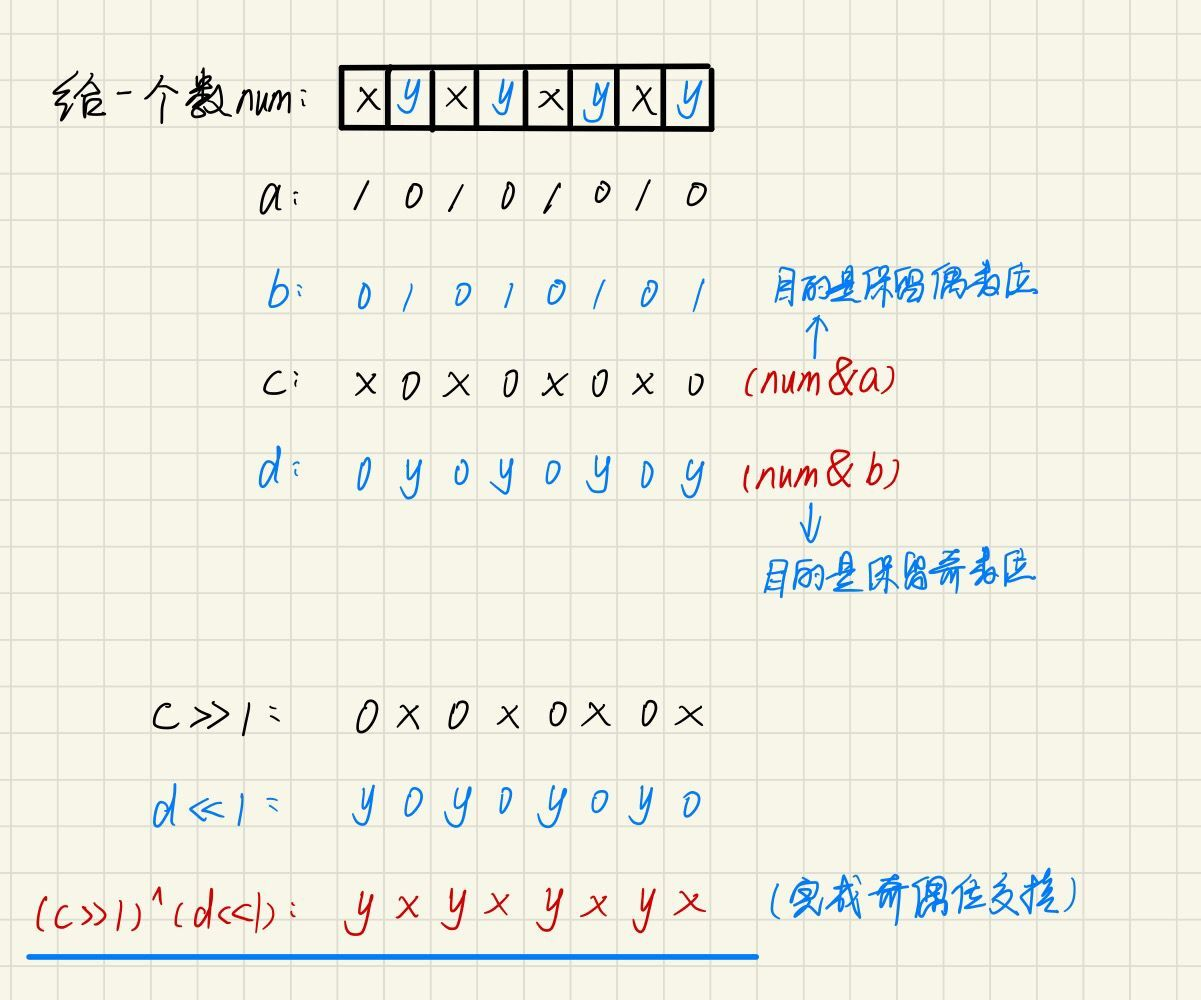``````#include<stdio.h>int main(){	int num = 9; //0000....00001001	int a = 0xaaaaaaaa;  //1010....10101010	int b = 0x55555555;  //0101....01010101	int c = num & a;  //目的是保留num的偶数位	int d = num & b;  //目的是保留num的奇数位 	printf("%%%%d
", ((c >> 1) ^ (d << 1))); 	return 0;}``````

# 五、笔者请求